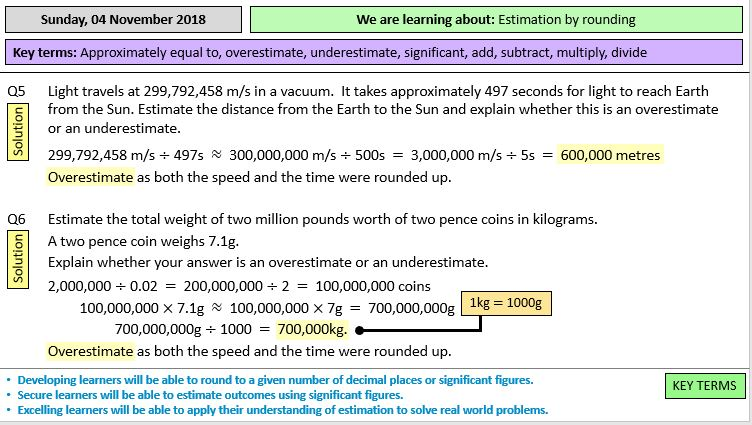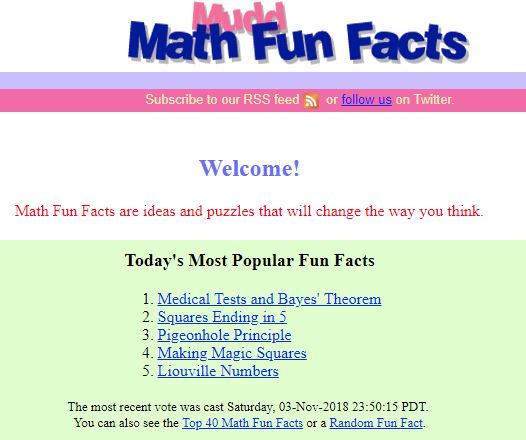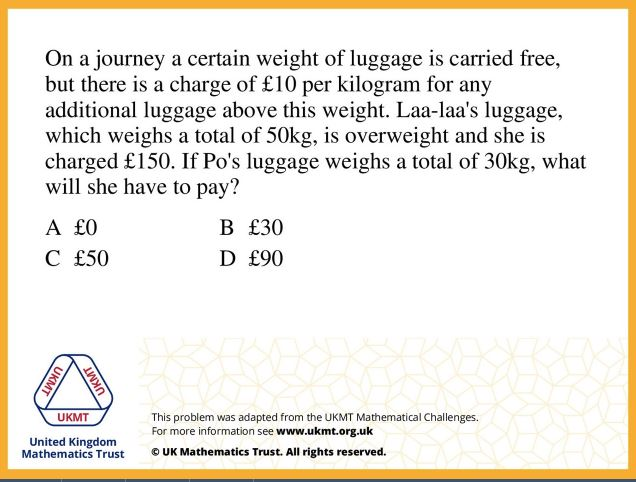# No Calculators Allowed…

Working with a student recently taking her university admission test for Medicine, I have been looking at some BMAT questions. The practice papers with explained answers provide a good understanding of the test format, different question types and skills assessed by the BMAT examination.

There is no doubt that, given the time pressure, students need to be skilled at performing calculations by hand. The fact that questions are in multiple-choice format is helpful, skills in estimation will clearly be an important strategy in answering questions requiring any calculations.

Even though students have to do one GCSE paper without a calculator, calculators are allowed in all A Level Mathematics and Science exams; looking at these BMAT questions made me wonder if we put the calculators aside enough.

This has prompted me to look again at good resources for practising these important skills of estimation and doing calculations by hand.Estimation by rounding by cparkinson3 is is a complete lesson providing a great variety of problems from basic skills to more challenging ones where learners have to apply their understanding of estimation to solve real-world problems. All resources needed are provided.

On Transum Mathematics you will find many estimation resources including Rough Answers illustrated here. Note the different levels available.

Mathsbot resources give us the opportunity to select non-calculator questions. A revision grid, with such questions, is a good example.

A starter is often a good opportunity to look at strategies for quick calculation, I have used several from Mudd Math Fun Facts which is a site well worth exploring if you are not familiar with it.Questions such as this can make a great starter for a lesson and provide the chance to discuss number operations and the relationships between them. Manipulating numbers like this can also help with algebraic manipulation.

Looking for some more examples of this type, I came across a really useful resource on TES, “If I know this then I also know …” by Piers Butler. This would make an ideal lesson starter. As it is an Excel spreadsheet, I thought it would be simple to add another worksheet with the answers and created the Excel file CY If_I_know_this_then_I_also_know_ which is a copy of the original, but just adds another worksheet with the answers.

Thank you Piers! I have added this to the Number collection on Mathematics Starters where you will find other ideas for Number starters.UK Maths Challenge questions provide an opportunity for working without a calculator. Maths Challenge questions are an excellent resource at any time – not just for preparation for the competitions, particularly with the increased requirement for problem solving skills at all levels. On the outstanding Diagnostic Questions site, you can choose Junior Maths Challenge questions by topic by choosing the Themed Quizzes option. These quizzes consist of sets of four or five questions grouped by topic. (Log in to Diagnostic Questions to use the link.)

Further Resources
Wikipedia mental calculation

Mental Arithmetic Training site